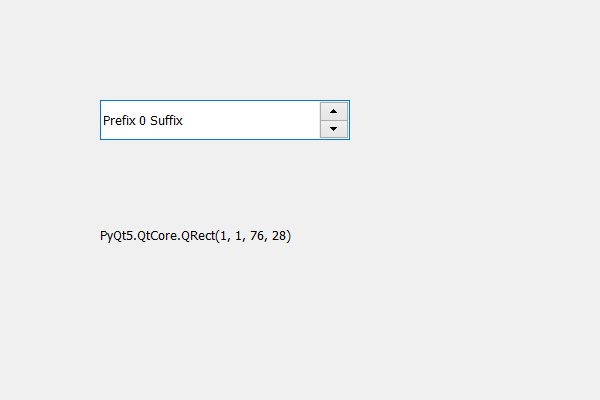Related Articles

# PyQt5 QSpinBox – Getting Children Rectangle

• Last Updated : 17 May, 2020

In this article we will see how we can get the children rectangle, children rectangle is the bounding rectangle of the spin box’s children. In order to do this we use `childrenRect` method. If we delete all the children of the spin box then this property contains a rectangle with zero width and height located at the origin.

In order to do this we use childrenRect method.

Syntax : spin_box.childrenRect()

Argument : It takes no argument

Return : It returns QRect object

Note : Hidden children are excluded

Below is the implementation

 `# importing libraries``from` `PyQt5.QtWidgets ``import` `*` `from` `PyQt5 ``import` `QtCore, QtGui``from` `PyQt5.QtGui ``import` `*` `from` `PyQt5.QtCore ``import` `*` `import` `sys`` ` ` ` `class` `Window(QMainWindow):`` ` `    ``def` `__init__(``self``):``        ``super``().__init__()`` ` `        ``# setting title``        ``self``.setWindowTitle(``"Python "``)`` ` `        ``# setting geometry``        ``self``.setGeometry(``100``, ``100``, ``600``, ``400``)`` ` `        ``# calling method``        ``self``.UiComponents()`` ` `        ``# showing all the widgets``        ``self``.show()`` ` `        ``# method for widgets``    ``def` `UiComponents(``self``):``        ``# creating spin box``        ``self``.spin ``=` `QSpinBox(``self``)`` ` `        ``# setting geometry to spin box``        ``self``.spin.setGeometry(``100``, ``100``, ``250``, ``40``)`` ` `        ``# setting prefix to spin``        ``self``.spin.setPrefix(``"Prefix "``)`` ` `        ``# setting suffix to spin``        ``self``.spin.setSuffix(``" Suffix"``)`` ` `        ``# creating a label``        ``self``.label ``=` `QLabel(``"Label "``, ``self``)`` ` `        ``# setting geometry to the label``        ``self``.label.setGeometry(``100``, ``200``, ``200``, ``70``)`` ` `        ``# getting the children rectangle``        ``children_rectangle ``=` `self``.spin.childrenRect()`` ` `        ``# setting text to the label``        ``self``.label.setText(``str``(children_rectangle))`` ` `        ``# making label multi line``        ``self``.label.setWordWrap(``True``)`` ` ` ` `# create pyqt5 app``App ``=` `QApplication(sys.argv)`` ` `# create the instance of our Window``window ``=` `Window()`` ` `# start the app``sys.exit(App.``exec``())`

Output :Attention geek! Strengthen your foundations with the Python Programming Foundation Course and learn the basics.

To begin with, your interview preparations Enhance your Data Structures concepts with the Python DS Course. And to begin with your Machine Learning Journey, join the Machine Learning – Basic Level Course

My Personal Notes arrow_drop_up